Next: Eventual Byzantine Agreement Up: Byzantine Failures in Previous: Written Messages

## Optimizing Byzantine Agreement

The Byzantine Generals Problem is a paradigm for distributed processes, some of which may be faulty. It might be fair to assume that most of the time, all processes will be correct (i.e., not faulty). An easy way to reduce the number of messages sent in a Byzantine Agreement algorithm would be to not run the algorithm at all if all of the processes are correct. We should then develop a Fault Detection algorithm which we run first, which would be weaker than the Byzantine Agreement algorithm. Specifically, either all processes are correct and they should come to a concensus, or at least one should detect the presence of a faulty process. Once a faulty process is detected, then the full-blown Byzantine Agreement algorithm can be run.

Hadzilacos and Halpern  showed that with oral messages, an average of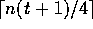messages are necessary for such an algorithm, where n is the number of processes and t is the number of expected faulty processes. With written messages, an average of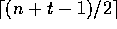messages are necessary, which is considerably better.

Instead of considering the number of messages, we could consider the number of rounds. An algorithm which tolerates t faulty processes must run at least t+1 rounds in worse-case execution; however, if the actual number of faults f is less than t, then there may be a way for the algorithm to terminate earlier. This is referred to as early stopping, and was described by Dolev et al. in . They show that if only fail-stop failures are allowed, then the minimum number of rounds is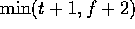, and they present an algorithm that achieves this bound. Since Byzantine failures are not considered, this result is somewhat weaker, but possibly useful in some systems where crashes are guarenteed to be the only faults.

Another approach is to use non-determinancy (i.e., probability). In , Bracha presents a non-deterministic algorithm with an expected number of rounds of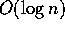, as long as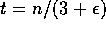for oral messages or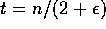for written messages. (The technique was later modified to yield a protocol with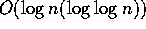expected rounds.)

The key is to use other Byzantine Agreement algorithms which have the following propreties: If t (number of faulty processes) is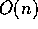then the algorithm is expenential in n, but if t is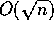then the expected number of rounds is constant. (There are several such algorithms, and our meta-algorithm will work with any ``plug-in'' algorithm with the above propreties.) If we can convert a system of n processes andfaulty processes into one with m processes and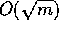faulty processes, then we can solve this new problem in constant time, leaving us only with the time to translate one system into the other.

Bracha shows that if we choose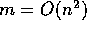and have each of these m processes be compound processes of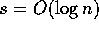actual processes (each of which may be in several compound processes), then we can choose the compound processes in such a way that at most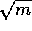of them are faulty. A standard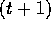-round Byzantine Agreement protocol can be run in each of the m compound processes (with an expectednumber of rounds), and the result can be run on the m compound processes, with an expected constant number of rounds. This yields a total expected number of rounds of.Next: Eventual Byzantine Agreement Up: Byzantine Failures in Previous: Written Messages

Lawrence Kesteloot
Fri Jan 20 16:38:32 EST 1995